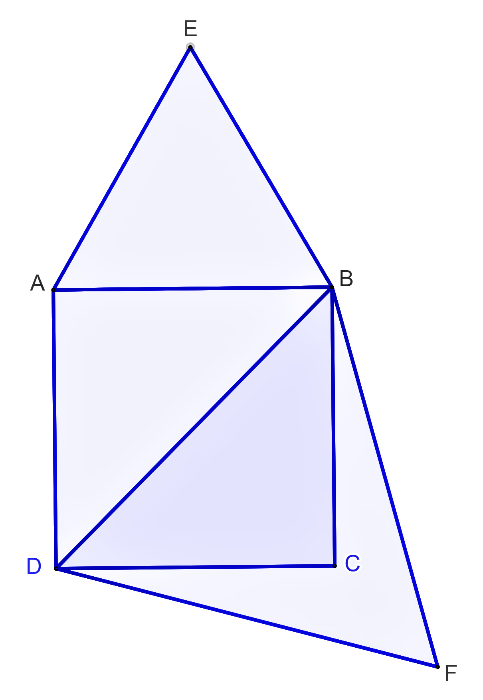# Ex.6.4 Q7 Triangles Solution - NCERT Maths Class 10

Go back to  'Ex.6.4'

## Question

Prove that the area of an equilateral triangle described on one side of a square is Equal to half the area of the equilateral triangle described on one of its diagonals.## Text Solution

Reasoning:

As we know that the ratio of the areas of two similar triangles is equal to the square of the ratio of their corresponding sides.

Steps:

$$\Delta ABE\,\,$$ is described on the side $$AB$$ of the square $$ABCD$$

$$\Delta DBF$$ is described on the diagonal $$BD$$ of the square $$ABCD$$

Since $$\Delta ABE\,$$ and $$\,\Delta DBF$$ are equilateral triangles

$$\Delta ABE \sim \Delta DBF$$      [each angle in an equilateral triangle measures $${{60}^ \circ}$$ ]

The ratio of the areas of two similar triangles is equal to the square of the ratio of the corresponding sides.

\begin{align}\frac{{Area\,\,of\,\,\Delta ABE}}{{Area\,\,of\,\,\Delta DBF}}&= \frac{{{{(AB)}^2}}}{{{{(DB)}^2}}} \\\frac{{Area\,\,of\,\,\Delta ABE}}{{Area\,\,of\,\,\Delta DBF}}&= \frac{{{{\left( {AB} \right)}^2}}}{{{{\left( {\sqrt 2 AB} \right)}^2}}}\end{align}   [$$\because$$ diagonal of a square is $$\,\sqrt 2\times$$ side]

\begin{align}\frac{{Area\,of\,\Delta ABE}}{{Area\,of\,\Delta DBF}}&= \frac{{A{B^2}}}{{2A{B^2}}}\\\frac{{Area\,of\,\Delta ABE}}{{Area\,of\,\Delta DBF}} &= \frac{1}{2}\\ \Rightarrow \quad \text{Area of }\Delta ABE &= \frac{1}{2} \text{Area of }\Delta DBF\end{align}

Tick the correct answer and justify.

Learn from the best math teachers and top your exams

• Live one on one classroom and doubt clearing
• Practice worksheets in and after class for conceptual clarity
• Personalized curriculum to keep up with school